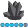## Help!

I have patient encounter file with more than 13000 records. File structure is like this.

No  Problemtype1     problemtype1b    problemtype1c  ...  problemtype49   prblemtype49c   outcome1   outcome1b ...  outcome49c

--------------------------------------------------------------------------------------------------------------------------------------------------------------

1          aa                            bb                         cc                       aa                 bb                   solved          rejected           denied

2

...

13000

I need to count respective outcomes if problemtype is 'aa'. I tried using 2 dimentional array but its not working.

6 REPLIES 6

## Re: Help!

So if any problem type is 'aa' you need to summarize the different values of outcome variables? How many problemtype variables and outcome and what are there naming conventions? Does the solution from @PGStats work for you from the other question you posted?

## Re: Help!

No. I am getting error.

ERROR: Array subscript out of range at  problemType = pt{i};

## Re: Help!

You didn't answer any of my questions...
Is the rule if any problemtype variable is equal to 'aa' you need to summarize the different variables?
How many problemtype variables?
What naming conventions are used?

Can you simplify the problem to say 10 problemtype variables and 4 observations and show your expected input and output?

## Re: Help!

I am sorry. I was so much into solving problem.

There are 147 problemtype variables and 147 Outcome variables. I also tried to simplify and run code. With minor change in solution given by PGstats, I solved problem.

Thanks a lot everyone for inputs.

## Re: Help!

@BKPatel wrote:

No. I am getting error.

ERROR: Array subscript out of range at  problemType = pt{i};

Means that you assigned a value to i that doesn't match the size of the array PT when created. Usually by incrementing to large but if your base index is something other than 1 then othe possibilities abound.Jim_G
Pyrite | Level 9

## Re: Help!

Here is some sample code. change the var and arrays from 5 to 49.

data have; *infile yourfile end=eof;
input n:5. (prob1 prob2 prob3 prob4 prob5) (:\$8.)
(outcom1 outcom2 outcom3 outcom4 outcom5) (:\$20.);

array prob {5} \$8 prob1-prob5;
array outcom {5} \$20 outcom1-outcom5;
do x=1 to 5 ;
if prob{x}='aa' then do;
if outcom{x}='solved' then solved+1;
if outcom{x}='rejected' then rejected+1;
if outcom{x}='denied' then denied+1;
end; end;
*if eof then output;
cards;
1 aa bb cc aa bb solved rejected denied solved denied
2 aa bb cc aa bb solved rejected denied solved denied
3 aa bb cc aa bb solved rejected denied solved denied
;
proc print; run;

Discussion stats
• 6 replies
• 953 views
• 0 likes
• 4 in conversation